Finding cube root by prime factorisation method

Let’s do this by examples

### Find cube root of 216?

Let’s do prime factorization of 216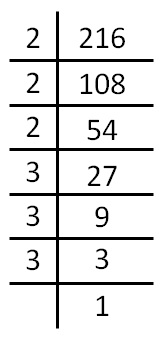Thus,

216  = 2 × 2 × 2 × 3 × 3 × 3

Now,

We make groups of 3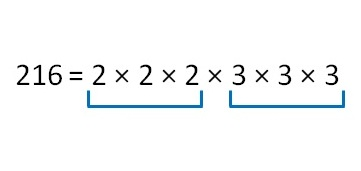Therefore,

Cube root of 216 = 2 × 3

= 6

### Find cube root of 729?

Doing Prime factorization of 729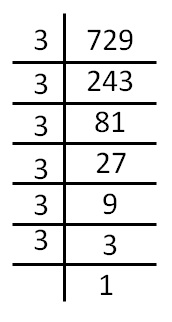We see that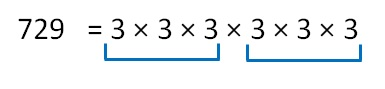Therefore,

Cube root of 729 = 3 × 3

= 9

### Find cube root of 3375?

Doing Prime factorization of 3375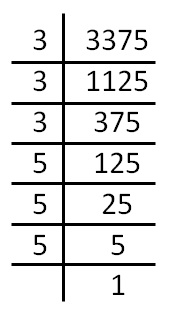We see that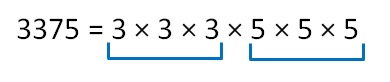Therefore,

Cube root of 3375 = 3 × 5

= 15

### Find cube root of 6859?

Doing Prime factorization of 6859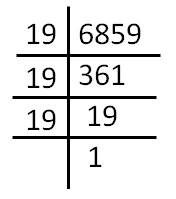We see that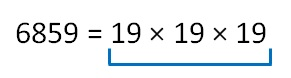Therefore,

Cube root of 6859 = 19

### Find cube root of 10648?

Doing Prime factorization of 10648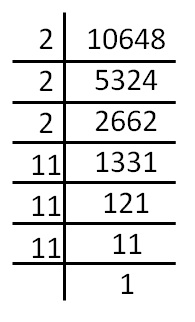We see that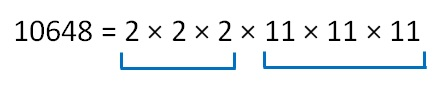Therefore,

Cube root of 10648 = 2 × 11

= 22

### Find cube root of 74088?

Doing Prime factorization of 74088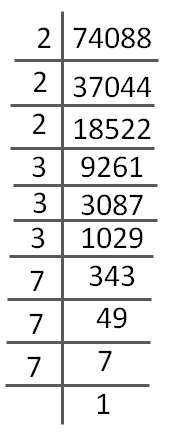We see that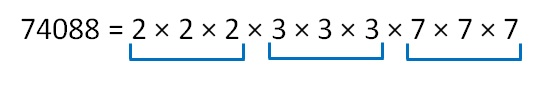Therefore,

Cube root of 74088 = 2 × 3 × 7

= 42

Learn in your speed, with individual attention - Teachoo Maths 1-on-1 Class